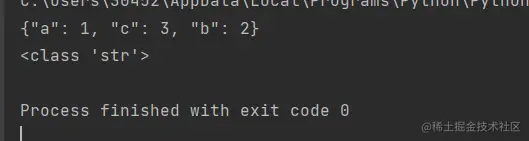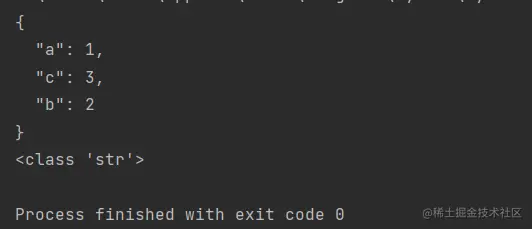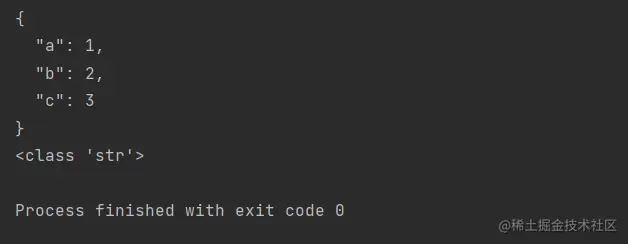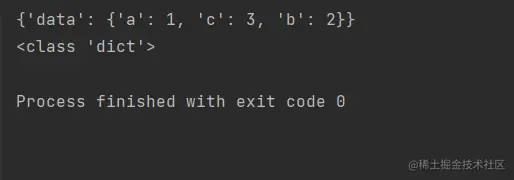# Use of Python JSON module

2022-02-02 04:54:53

# Python JSON Use of modules

## dumps(　) take Python Data transformation JSON Format

Conversion correspondence table

Python JSON
dict object
list,tuple array
str,unicode string
int,float,long number
True true
False false
None null
``````import json
li={'a':1,'c':3,'b':2}
print(json.dumps(li))
print(type(json.dumps(li)))
Copy code ``````### Set indent indent

``````import json
li={'a':1,'c':3,'b':2}
print(json.dumps(li,indent=2))
print(type(json.dumps(li)))
Copy code ``````### Sort sort_keys

``````import json
li={'a':1,'c':3,'b':2}
print(json.dumps(li,sort_keys=True,indent=2))
print(type(json.dumps(li)))
Copy code ``````## loads(　) take JSON Format data into Python data

Conversion correspondence table

JSON Python
object dict
array list
string unicode
number(int) int,long
number(real) float
trun Trun
false False
null None
``````import json
li={'data':{'a':1,'c':3,'b':2,}}
a=json.dumps(li)